# Categorical predictors

11/16/2020

## Instructions:

Use the left and right arrow keys to navigate the presentation forward and backward respectively. You can also use the arrows at the bottom right of the screen to navigate with a mouse.

FAIR USE ACT DISCLAIMER:
This site is for educational purposes only. This website may contain copyrighted material, the use of which has not been specifically authorized by the copyright holders. The material is made available on this website as a way to advance teaching, and copyright-protected materials are used to the extent necessary to make this class function in a distance learning environment. The Fair Use Copyright Disclaimer is under section 107 of the Copyright Act of 1976, allowance is made for “fair use” for purposes such as criticism, comment, news reporting, teaching, scholarship, education and research.

## Outline

• The following topics will be covered in this lecture:
• A review of binary categorical predictors
• Factors with more than two levels
• Alternative encoding
• Models with only categorical predictors
• Diagnostics
• Models with multiple factors

## A review of binary categorical predictors

• In many circumstances we have been using categorical predictors in which there are one or more categories, e.g.,
library(faraway)
str(teengamb)

'data.frame':   47 obs. of  5 variables:
$sex : int 1 1 1 1 1 1 1 1 1 1 ...$ status: int  51 28 37 28 65 61 28 27 43 18 ...
$income: num 2 2.5 2 7 2 3.47 5.5 6.42 2 6 ...$ verbal: int  8 8 6 4 8 6 7 5 6 7 ...
gamble: num 0 0 0 7.3 19.6 0.1 1.45 6.6 1.7 0.1 ...  • Because the category of “M” or “F” have no mathematical meaning in the regression, this has become coded as a 1 or 0 in the model. • The terminology for this encoding of a category as an integer value is commonly known as a “dummy variable” where the value itself doesn't have a specific meaning, but the parameter will describe the effect. • We have seen the effect of this this coding a giving the option of a “switch” on or off of a parameter $$\beta_{sex}$$, which adjusts the model for the categorical level. • Particularly, this gives an adjusted intercept term when it is “turned on” with a value of a 1, or the original intercept $$\beta_0$$ when the value of the category is 0. • There are, however, several approaches we could take for a categorical variable as before with two levels… ### A review of binary categorical predictors • For instance, supposing we model the response $$Y$$ (gamble) in terms of a continuum variable $$X$$ (income) and a category (sex) with $$d\in\{0,1\}$$, we can neglect the categorical “switch” in the case we don't find it to be useful in the analysis. \begin{align} Y = \beta_0 + \beta_1 X + \epsilon. \end{align} sumary(lm(gamble ~ income, data=teengamb))   Estimate Std. Error t value Pr(>|t|) (Intercept) -6.3246 6.0299 -1.0489 0.2998 income 5.5205 1.0358 5.3298 3.045e-06 n = 47, p = 2, Residual SE = 24.94821, R-Squared = 0.39  par(mai=c(1.5,1.5,.5,.5), mgp=c(3,0,0)) plot(gamble ~ income, teengamb, cex=3, cex.lab=3, cex.axis=1.5) abline(-6.3246, 5.5205)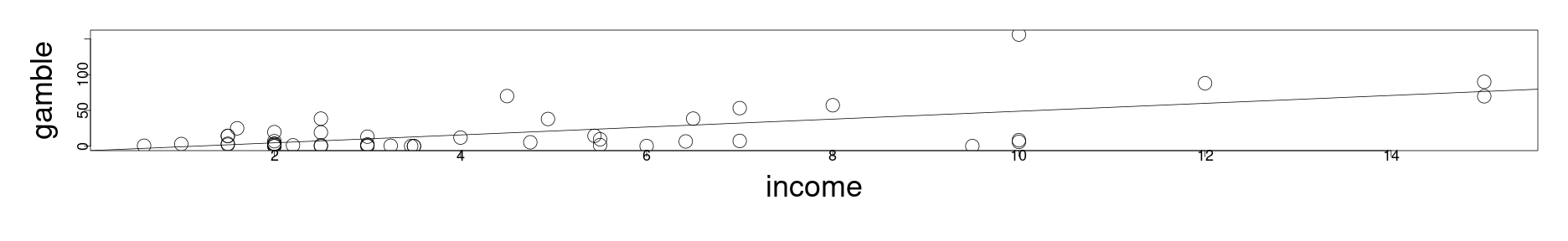### A review of binary categorical predictors • On the other hand, we might find that the continuum variable is not useful in the analysis. • In this case, we can model the response purely in terms of the “switch” part of the equation": \begin{align} Y = \beta_0 + \beta_2 d + \epsilon \end{align} so that we describe the response in terms of variation around two intercept levels. sumary(lm(gamble ~ sex, data=teengamb))   Estimate Std. Error t value Pr(>|t|) (Intercept) 29.7750 5.4983 5.4153 2.282e-06 sex -25.9092 8.6477 -2.9961 0.004437 n = 47, p = 2, Residual SE = 29.09414, R-Squared = 0.17  par(mai=c(1.5,1.5,.5,.5), mgp=c(3,0,0)) plot(gamble ~ sex, teengamb, cex=3, cex.lab=3, cex.axis=1.5) points(list(x=c(0,1), y=c(29.775, 29.775-25.9092)), pch=17, cex=3)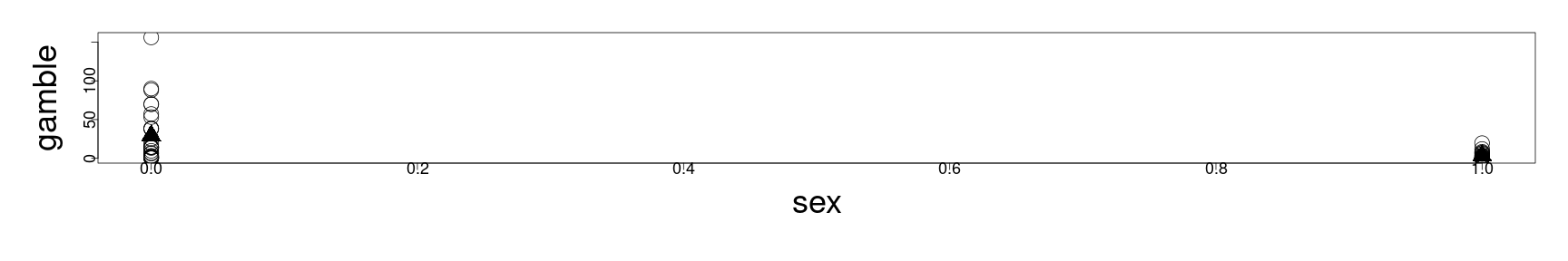### A review of binary categorical predictors • We can also consider modeling the response with both the continuum variable and the categorical variable, where the slope of the response in terms of the continuum variable will be the same for each category; • in this case $$\beta_{sex}$$ describes the distance between the two regression lines (the adjusted intercept): \begin{align} Y = \beta_0 + \beta_1 X + \beta_2 d + \epsilon \end{align} sumary(lm(gamble ~ income + sex, data=teengamb))   Estimate Std. Error t value Pr(>|t|) (Intercept) 4.04083 6.39435 0.6319 0.530698 income 5.17158 0.95105 5.4378 2.245e-06 sex -21.63439 6.80880 -3.1774 0.002717 n = 47, p = 3, Residual SE = 22.75428, R-Squared = 0.5  par(mai=c(1.5,1.5,.5,.5), mgp=c(3,0,0)) plot(gamble ~ income, teengamb, cex=3, cex.lab=3, cex.axis=1.5) abline(4.04083, 5.17158) abline(4.04083-21.63429, 5.17158)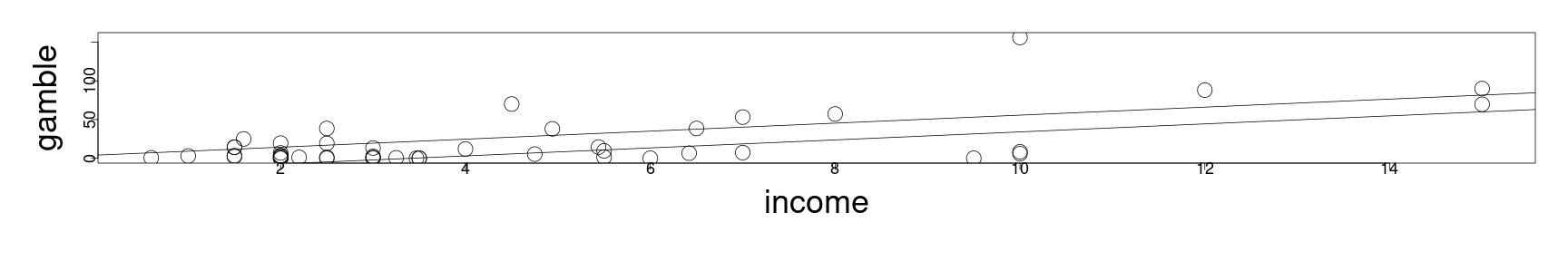### A review of binary categorical predictors • But it may also make sense to model the response with separate slopes and separate intercepts, done in R with sumary(lm(gamble ~ income * sex, data=teengamb))   Estimate Std. Error t value Pr(>|t|) (Intercept) -2.65963 6.31642 -0.4211 0.675804 income 6.51812 0.98808 6.5967 4.951e-08 sex 5.79960 11.20025 0.5178 0.607245 income:sex -6.34320 2.14456 -2.9578 0.005018 n = 47, p = 4, Residual SE = 20.98167, R-Squared = 0.59  par(mai=c(1.5,1.5,.5,.5), mgp=c(3,0,0)) plot(gamble ~ income, teengamb, cex=3, cex.lab=3, cex.axis=1.5) abline(-2.65963, 6.51812) abline(-2.65963+5.79960, 6.51812-6.34320)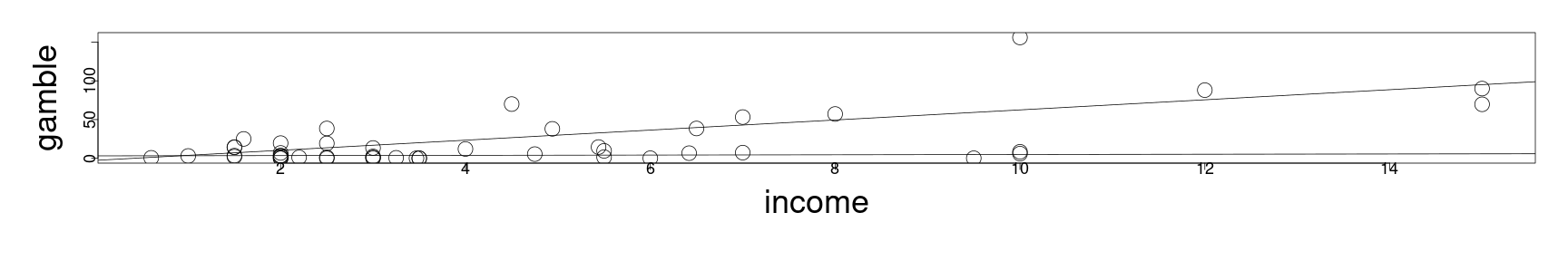### A review of binary categorical predictors • This can be seen in a functional form as a model like: \begin{align} Y = \beta_0 + \beta_1 X + \beta_2 d + \beta_{x:d}X \times d + \epsilon \end{align} • Here the parameter $$\beta_{x:d}$$ is activated to adjust the slope when $$d=1$$ in the same way that the intercept is adjusted for $$d=1$$. • This parameter is commonly known as the interaction term, which can be more difficult to interpret at times; • loosely this is the slope of the response in terms of the variable $$X$$ conditioned on the category. • This will also generally extend to multiple predictors, where the category will shift the slope or intercept as determined optimal by the least squares solution. • The interaction term can be an important element in our analysis, as it is seen in the gambling data, the relationship between income and gambling is almost flat for women. • Performing the model fitting as above is a formal way of computing two separate models (and their differences) simultaneously when it appears that there is reason for two separate models. • In particular, this is appropriate in the case when we don't think that the difference between the trend in the two categories should be constant. ### A review of binary categorical predictors • Generally speaking it is a good idea to perform some exploratory analysis with respect to the categorical variable, to determine if there is a reason to model these separately. par(cex=2, mai=c(1.5,1.5,.5,.5), mgp=c(1,0,0)) boxplot(gamble ~ sex, teengamb)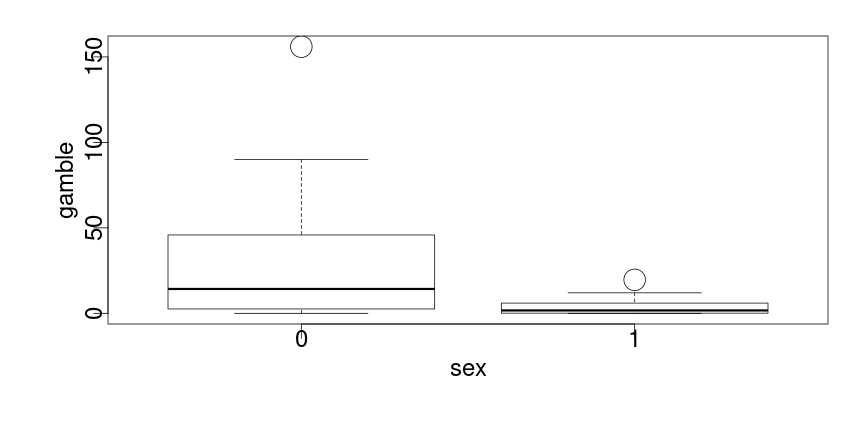• From the box plots, we have a strong indication that the sub-populations differ in terms of their means and variances. • We may wish to formalize this somewhat with a two-way t-test, but in this case we should be aware of the issue of the Gaussian distribution assumption. • Particularly, both have strong indications of non-normal data, even with outliers removed. ### A review of binary categorical predictors • In the case of normal data, or large sample sizes using the central limit theorem, we can appeal to a two-sample t-test to check for the differences of means. • Likewise, if the data is normal, we can appeal to an F-test to check the differences in the variances; • however, the F-test is quite sensitive to departures from normality. • For the teengamb data, neither the normality nor the large sample size assumption hold and therefore we can appeal at least to the Kolmolgorov-Smirnov test for the continuous response variable: gamb_m <- teengamb[teengambsex==0,]
gamb_m <- gamb_m[gamb_m$gamble<120,] gamb_f <- teengamb[teengamb$sex==1,]
ks.test(gamb_m$gamble, gamb_f$gamble)


Two-sample Kolmogorov-Smirnov test

data:  gamb_m$gamble and gamb_f$gamble
D = 0.50292, p-value = 0.007095
alternative hypothesis: two-sided

• As was noted clearly by visual inspection, the two sub-samples do appear to be drawn from statistically distinct sub-populations.

• This is corroborated by our analysis in the activities, where we also saw unequal variances in the residuals with respect to the individually subset models.

## An example with heating efficiency

• A homeowner in England recorded his weekly natural gas consumption, in thousands of cubic feet, during two winter heating seasons.

library(MASS)
library(ggplot2)
ggplot(aes(x=Temp,y=Gas),data=whiteside)+geom_point()+facet_grid(~
Insul)+geom_smooth(method="lm")+ theme(axis.text=element_text(size=20), axis.title=element_text(size=20,face="bold"), strip.text.x = element_text(size = 20, face="bold"))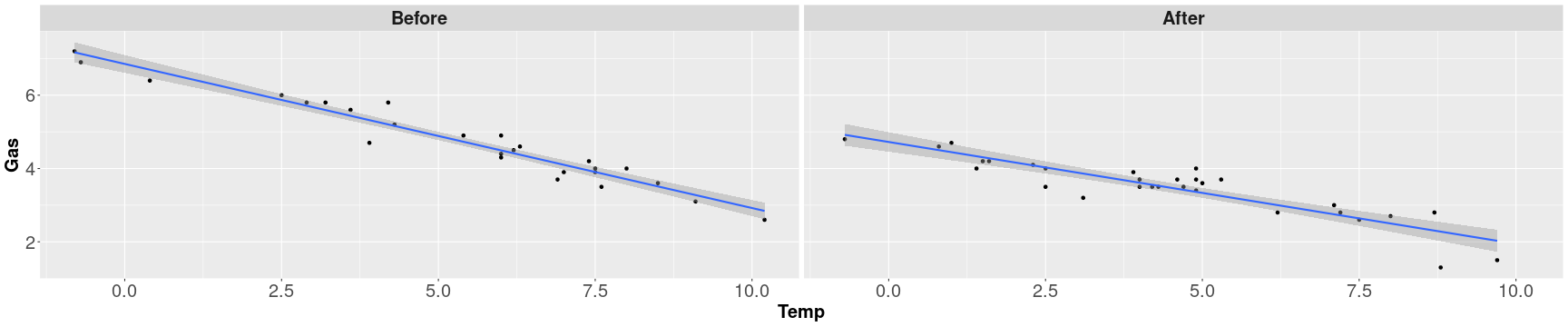• The horizontal axis is “probably the weekly average, daily minimum temperature” (see help file).

### An example with heating efficiency

• The previous plots, there is less gas used after the additional insulation is added, but the difference varies by temperature.

• Producing the multiple models with interaction, we have

lmod <- lm(Gas ~ Temp*Insul, whiteside)
sumary(lmod)

                 Estimate Std. Error  t value  Pr(>|t|)
(Intercept)      6.853828   0.135964  50.4091 < 2.2e-16
Temp            -0.393239   0.022487 -17.4874 < 2.2e-16
InsulAfter      -2.129978   0.180092 -11.8272 2.316e-16
Temp:InsulAfter  0.115304   0.032112   3.5907 0.0007307

n = 56, p = 4, Residual SE = 0.32300, R-Squared = 0.93

• Here we see that the pre-insulation change in gas consumption is approximately .393 cubic feet per 1 degree change in temperature, whereas after the insulation it is approximately 0.278 cubic feet.

• Producing separate slopes and intercepts does improve the overall fit of the model in this case.
• That is, it is shown in the summary that the interaction term is significant.
• However, some additional care should be taken with the intercept terms, representing the ammount of gas consumption when the temperature is zero.

• Here there are very few observations, and therefore the uncertainty of these parameters representing the zero-temperature consumption will naturally be higher.

### An example with heating efficiency

• Generally, if the range or center of mass of the predictors is far away from zero, we can rectify this issue by re-centering the variable at its mean (computing the anomalies).

• By centering at the mean, the intercept will actually describe the predicted value for the mean and the change in the predictor describes the change as the variable deviates from the mean.

mean(whiteside$Temp)   4.875  whiteside$ctemp <- whiteside$Temp - mean(whiteside$Temp)
lmodc <- lm(Gas ~ ctemp*Insul, whiteside)
sumary(lmodc)

                  Estimate Std. Error  t value  Pr(>|t|)
(Intercept)       4.936788   0.064241  76.8485 < 2.2e-16
ctemp            -0.393239   0.022487 -17.4874 < 2.2e-16
InsulAfter       -1.567872   0.087713 -17.8750 < 2.2e-16
ctemp:InsulAfter  0.115304   0.032112   3.5907 0.0007307

n = 56, p = 4, Residual SE = 0.32300, R-Squared = 0.93

• In this case, re-centering the model gives a more natural interpretation.

### An example with heating efficiency

• To obtain the model back in the normal coordinates, we can make a change of variables that includes this shift:

\begin{align} &Y = \beta_0 + \beta_{ctemp} \left( X- 4.875\right) + \beta_{InsulAfter} d + \beta_{ctemp:InsulAfter} X \times d\\ \Leftrightarrow & Y = 4.936788 - 0.393239 \left( X - 4.875\right) + -1.567872 d + 0.115304 X \times d \\ \Leftrightarrow & Y = 6.853828 - 0.393239X + -1.567872 d + 0.115304 X \times d \end{align}

### An example with heating efficiency

• Whereby plotting, we find:
par(mai=c(1.5,1.5,.5,.5), mgp=c(3,0,0),usr=c(-2,12, 0, 8))
plot(Gas ~ Temp, whiteside[whiteside$Insul == "Before",], cex=3, cex.lab=3, cex.axis=1.5) abline(6.853828, -0.393239)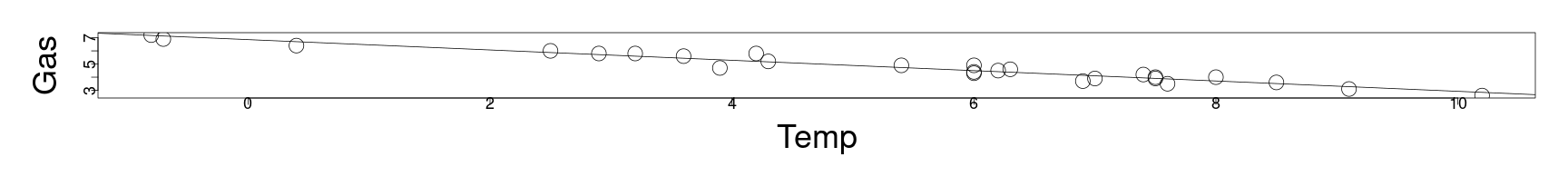par(mai=c(1.5,1.5,.5,.5), mgp=c(3,0,0), usr=c(-2,12, 0, 8)) plot(Gas ~ Temp, whiteside[whiteside$Insul == "After",], cex=3, cex.lab=3, cex.axis=1.5)
abline(6.853828-1.567872, -0.393239 + 0.115304)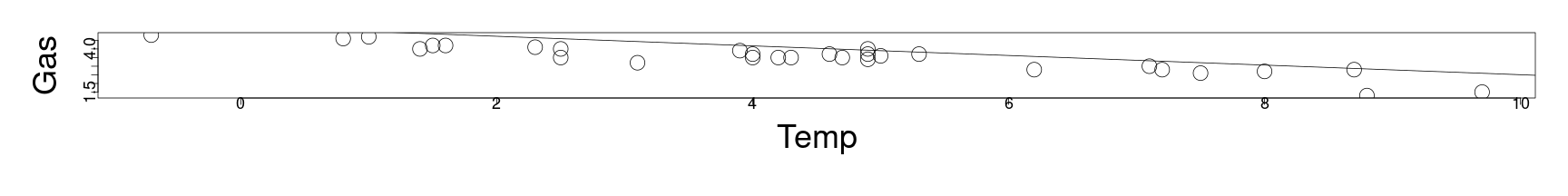## Factors with more than two levels

• We can consider how to generalize this approach to where there are multiple levels in a categorical variable.

• For instance, if we wanted to predict the price of a car, we might include several different color levels in a color categorical variable.
• If there are $$f$$ different levels, then we can create $$f-1$$ dummy variables $$\{d_2,\cdots, d_f\}$$ with the following meaning:

\begin{align} d_i = \begin{cases} 0 & \text{if case is not level } i\\ 1 & \text{if case is level } i \end{cases} \end{align}

• When a case contains a value that isn't level $$i=2,\cdots,f$$, then this automatically defaults to the level $$1$$, described by the default intercept term.
• This default intercept term is the reference level from which all other levels deviate.

• Cases self-identify if they belong to any other level with a 1 correspondingly in the dummy variable that corresponds to the appropriate level.
• We can consider multi-level factors in studying the longevity of fruitflies with respect to their sexual behaviors…

## Fruitfly example

• 125 male fruitflies are randomly divided into 5 groups of 25 each – the response variable will be the number of days the fruitflies live.

• The predictors for the response include thorax length, which is known to affect the longevity of the fly, and sexual activity.

• The groups are then studied with respect to one of several different environments:

1. one group is held without any female fruitflies;
2. one group is held with a single sexually active female fruitfly, per day;
3. one group is held with 8 sexually active female frutflies, per day;
4. a control group is held with a single non-sexually active female fruitfly, per day;
5. a final control group is held with 8 non-sexually active female fruitflies, per day.
• These groups are labeled “isolated”, “low”, “high”, “one” and “many” respectively.

### Fruitfly example

• Plotting all groups together, but distinguishing groups by symbols, we have:
par(mai=c(1.5,1.5,.5,.5), mgp=c(3,0,0))
plot(longevity ~ thorax, fruitfly, pch=unclass(activity), cex=3, cex.lab=3, cex.axis=1.5)
legend(0.63,100,levels(fruitfly\$activity),pch=1:5)